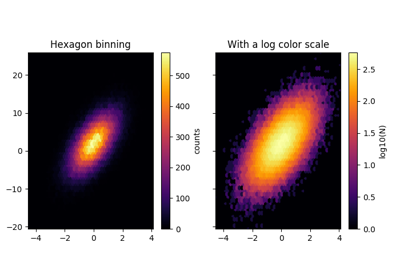matplotlib.axes.Axes.hexbin¶

Axes.hexbin(x, y, C=None, gridsize=100, bins=None, xscale='linear', yscale='linear', extent=None, cmap=None, norm=None, vmin=None, vmax=None, alpha=None, linewidths=None, edgecolors='face', reduce_C_function=<function mean>, mincnt=None, marginals=False, *, data=None, **kwargs)[source]

Make a hexagonal binning plot.

Make a hexagonal binning plot of x versus y, where x, y are 1-D sequences of the same length, N. If C is None (the default), this is a histogram of the number of occurrences of the observations at (x[i],y[i]).

If C is specified, it specifies values at the coordinate (x[i],y[i]). These values are accumulated for each hexagonal bin and then reduced according to reduce_C_function, which defaults to numpy's mean function (np.mean). (If C is specified, it must also be a 1-D sequence of the same length as x and y.)

Parameters: x, y : array or masked array C : array or masked array, optional, default is None gridsize : int or (int, int), optional, default is 100 The number of hexagons in the x-direction, default is 100. The corresponding number of hexagons in the y-direction is chosen such that the hexagons are approximately regular. Alternatively, gridsize can be a tuple with two elements specifying the number of hexagons in the x-direction and the y-direction. bins : {'log'} or int or sequence, optional, default is None If None, no binning is applied; the color of each hexagon directly corresponds to its count value. If 'log', use a logarithmic scale for the color map. Internally, $$log_{10}(i+1)$$ is used to determine the hexagon color. If an integer, divide the counts in the specified number of bins, and color the hexagons accordingly. If a sequence of values, the values of the lower bound of the bins to be used. xscale : {'linear', 'log'}, optional, default is 'linear' Use a linear or log10 scale on the horizontal axis. yscale : {'linear', 'log'}, optional, default is 'linear' Use a linear or log10 scale on the vertical axis. mincnt : int > 0, optional, default is None If not None, only display cells with more than mincnt number of points in the cell marginals : bool, optional, default is False if marginals is True, plot the marginal density as colormapped rectagles along the bottom of the x-axis and left of the y-axis extent : scalar, optional, default is None The limits of the bins. The default assigns the limits based on gridsize, x, y, xscale and yscale. If xscale or yscale is set to 'log', the limits are expected to be the exponent for a power of 10. E.g. for x-limits of 1 and 50 in 'linear' scale and y-limits of 10 and 1000 in 'log' scale, enter (1, 50, 1, 3). Order of scalars is (left, right, bottom, top). object a PolyCollection instance; use get_array() on this PolyCollection to get the counts in each hexagon. If marginals is True, horizontal bar and vertical bar (both PolyCollections) will be attached to the return collection as attributes hbar and vbar. cmap : object, optional, default is None a matplotlib.colors.Colormap instance. If None, defaults to rc image.cmap. norm : object, optional, default is None matplotlib.colors.Normalize instance is used to scale luminance data to 0,1. vmin, vmax : scalar, optional, default is None vmin and vmax are used in conjunction with norm to normalize luminance data. If None, the min and max of the color array C are used. Note if you pass a norm instance your settings for vmin and vmax will be ignored. alpha : scalar between 0 and 1, optional, default is None the alpha value for the patches linewidths : scalar, optional, default is None If None, defaults to 1.0. edgecolors : {'face', 'none', None} or color, optional If 'face' (the default), draws the edges in the same color as the fill color. If 'none', no edge is drawn; this can sometimes lead to unsightly unpainted pixels between the hexagons. If None, draws outlines in the default color. If a matplotlib color arg, draws outlines in the specified color.

Notes

The standard descriptions of all the Collection parameters:

Property Description
agg_filter a filter function, which takes a (m, n, 3) float array and a dpi value, and returns a (m, n, 3) array
alpha float or None
animated bool
antialiased or antialiaseds Boolean or sequence of booleans
array ndarray
capstyle unknown
clim a length 2 sequence of floats; may be overridden in methods that have vmin and vmax kwargs.
clip_box a Bbox instance
clip_on bool
clip_path [(Path, Transform) | Patch | None]
cmap a colormap or registered colormap name
color matplotlib color arg or sequence of rgba tuples
contains a callable function
edgecolor or edgecolors matplotlib color spec or sequence of specs
facecolor or facecolors matplotlib color spec or sequence of specs
figure a Figure instance
gid an id string
hatch [ '/' | '\' | '|' | '-' | '+' | 'x' | 'o' | 'O' | '.' | '*' ]
joinstyle unknown
label object
linestyle or dashes or linestyles ['solid' | 'dashed', 'dashdot', 'dotted' | (offset, on-off-dash-seq) | '-' | '--' | '-.' | ':' | 'None' | ' ' | '']
linewidth or linewidths or lw float or sequence of floats
norm Normalize
offset_position [ 'screen' | 'data' ]
offsets float or sequence of floats
path_effects AbstractPathEffect
picker [None | bool | float | callable]
rasterized bool or None
sketch_params (scale: float, length: float, randomness: float)
snap bool or None
transform Transform
url a url string
urls List[str] or None
visible bool
zorder float

Note

In addition to the above described arguments, this function can take a data keyword argument. If such a data argument is given, the following arguments are replaced by data[<arg>]:

• All arguments with the following names: 'x', 'y'.

Examples using matplotlib.axes.Axes.hexbin¶Hexbin Demo